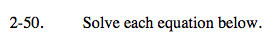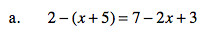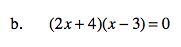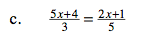### Home > CAAC > Chapter 14 > Lesson 14.2.1.4 > Problem2-50

2-50.Distribute the −1 to (x + 5) and solve.

2 − x − 5 = 7 − 2x + 3

x = 13Remember the Zero Product Property.

2x + 4 = 0; x − 3 = 0Multiply both sides by 15 to eliminate the denominators.

25x + 20 = 6x + 3